# Knowledge Base

## Solution 11807: Demonstrating Probability Distributions on the TI-83 Family, TI-84 Plus Family, and TI-Nspire™ Handheld in TI-84 Plus Mode Graphing Calculators.

### How can I compute the probability distributions in a binomial distribution on the TI-83 Family, TI-84 Plus Family or TI-Nspire Handheld in TI-84 Plus Mode?

The example below shows how to compute different probabilities in a binomial distribution.

Example:

The production of an electronic component has a large 20% defective rate. If a random selection of six components is taken,
1) What is the probability of getting exactly two defectives?
2) What is the probability of getting at most two defectives?
3) What is the probability of getting at least two defectives?
4) What is the probability of getting from two to four defectives?

Solution:

First, generate the complete distribution:.
1) Press [2nd][DISTR], select binompdf(.
2) Input 6, .2).
3) Press [STO->] L1.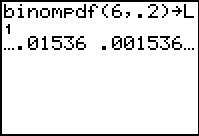4) Press [ENTER]. The distribution will be generated and stored in L1.
5) Enter the values 0 to 6 into L2, to clearly identify the different probabilities.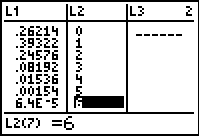Follow the steps below to solve the different questions.

Question 1:

The probability of getting exactly two defectives is P(1)=.39322. This value can be found in L1. To calculate it:
1) Press [2nd][DISTR], select binompdf(.
2) Input 6, .2, 1).
3) Press [ENTER].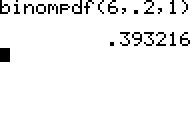Question 2:

The probability of getting at most two defectives is P(0)+ P(1)+P(2)=.90112. To calculate it directly:
1) Press [2nd][DISTR], select binomcdf(.
2) Input 6, .2, 2).
3) Press [ENTER].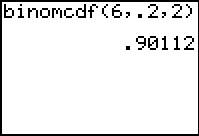Question 3:

The probability of getting at least two defectives is P(2)+P(3)+P(4)+P(5)+P(6)=1-(P(0)+P(1))= .34464. To calculate it:
1) Input 1-
2) Press [2nd][DISTR], select binomcdf(.
3) Input 6, .2, 1).
4) Press [ENTER].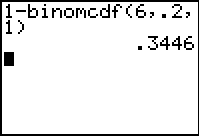Question 4:

The probability of getting from two to four defectives is P(2)+P(3)+P(4)=.34304 =(P(0)+P(1)+P(2)+ P(3)+P(4))-(P(0)+P(1)).To calculate it:
1) Press [2nd][DISTR], select binomcdf(.
2) Input 6, .2, 4)-
3) Press [2nd][DISTR], select binomcdf(.
4) Input 6, .2, 1).
5) Press [ENTER].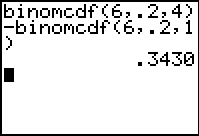The same result can be obtained by entering sum(binompdf(6, .2,{2,3,4}))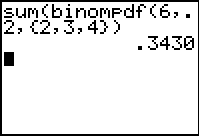Please see the TI-83 family and TI-84 Plus family guidebooks for additional information.

Please Note: TI-Nspire users may refer to the TI-84 Plus family guidebook.